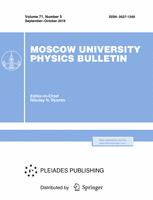Faculty of Physics
M.V.Lomonosov Moscow State University

# Mir-IR femtosecond laser pulses wavefront reconstruction using machine learning methods

## N. Y. Victorovich$^1$, A. V. Mitrofanov$^{2,3,4}$, M. V. Rozhko$^{1,3}$, N. G. Iroshnikov$^1$, D. A. Sidorov-Biryukov$^{1,3,4}$

### Memoirs of the Faculty of Physics 2023. N 4.

• Article
Annotation

The paper shows the possibility of using convolutional neural networks for a laser beam wavefront reconstruction by measuring the intensity distribution in the transverse plane at some distance behind the focusing system. The optimal distance from the focusing system to the measurement plane is determined at which the error for the rms devation of Zernike polynomials amplitudes decreases sharply. A neural network has been trained on a computer-generated samples of beams with various types of aberrations to reconstruct the wavefront of real beams in the presence of noise

Approved: 2023 September 22
PACS:
42.62.-b Laser applications
Authors
N. Y. Victorovich$^1$, A. V. Mitrofanov$^{2,3,4}$, M. V. Rozhko$^{1,3}$, N. G. Iroshnikov$^1$, D. A. Sidorov-Biryukov$^{1,3,4}$
$^1$\
$^2$\
$^3$\
$^4$National Research Center Kurchatov Institute### Moscow University Physics Bulletin### Science News of the Faculty of Physics, Lomonosov Moscow State University

This new information publication, which is intended to convey to the staff, students and graduate students, faculty colleagues and partners of the main achievements of scientists and scientific information on the events in the life of university physicists.